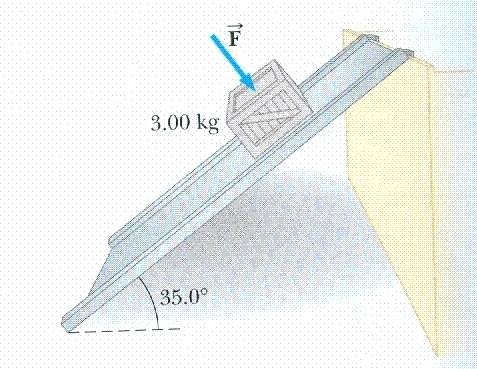# Finding perpendicular force on incline plane.

## Homework Statement

I'm having a lot of trouble trying to figure out this problem, any help would be great.
The coefficient of static friction between a 3 Kg box and a 35 degree incline ramp is 0.3. What minimum force F perpendicular to the incline must be applied to the box to prevent it from sidling down the incline?. Draw the free body diagram of the system. Show all your work.

Here is the free body diagram.## Homework Equations

part. b was find the minimum force F parallel.

## The Attempt at a Solution

I found out that the perpendicular force is = to the normal force. I used the sum of all forces to come up with N= mgcosθ. I also looked up some info on a different site and found this, which really confused me. I'm not quite sure where to take this.
u=.3
Fr=uN.
n-normal force
you need aply 55.39N of normal force.
parall force would be 16.863-.9=15.96.
working:
jus resolve the gravity force par&per to inclined plane.
0.9 is force that friction provoids.u can do it usin abv formula.
after resolvin force,u will find out force parallel to inc plane is 16.863.
so need 16.863-0.9 horizontal force.
in case of perpendicular force,
you need fig out what is the normal force which can make friction force equal's 16.863
16.863-.819=0.3*(X);

Let's keep this simple ,

As you have done I shall start by resolving everything parallel and perpendicular to the plane.

As show in the fig, the external force is F

On the incline, the Normal force will at act perpendicularly, and in this case will have to be equal to the total force exerted by the block on the planenin the perpendicular direction.

Hence, N = F + mgcos(35)

And now use frictional force = 0.3(N)

So then the parallel force would be N = F + mgsin(35)?, use 0.3(N)? what would be the total F? i see in the example it is 55.39n, im not getting that. Thanks alot!

For the parallel forces, mgsin(35) = friction.